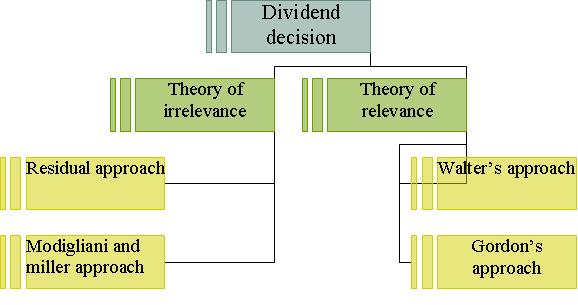# Divident policy, dividend decisions and valuation of shares

Dividend policy:

Dividend is the part of company’s profit which is given by company to its shareholders. As the shareholders invest their valuable money in the company, so they want to get the maximum return out of it. But in case the company pays the more of its earning in form of dividend than the company has to depend upon the outside resources for its survival. So it is necessary for the growth of the company to pay adequate amount of dividend.

Dividend decisions and valuation of shares:

It is generally said that the value of the firm is maximized if the shareholders get maximum wealth which means if they get maximum dividend. But some believe that the dividend decision does not affect the value of the firm. For this purpose the two theories have been given and these are discussed below.

1.) Theory of irrelevance: this theory states that the dividend decision does not affect the shareholders wealth as well as value of the firm. That’s why this theory is called theory of irrelevance. This theory further explained with the help of two different approaches.

i) Residual approach: as per this approach dividend decision does not affect the shareholders wealth as well as value of the firm. The investors don’t want to earn dividend only they want to earn high return on their investment. This means that if the firm is in the situation to gain high profit than the shareholders would like to keep their money with the firm and on the other hand if the firm is not in a position to get profitable opportunity than the shareholders would like to receive the earning in form of dividend.

ii) Modigliani and miller approach: as per this approach also dividend decision does not affect the shareholders wealth as well as value of the firm rather it is decided by the earning capacity of the firm or its investment policy. This theory assumes:

a) there are no taxes

b) there is a perfect market

c) investors act rationally

d) no transaction cost

e) all earning goes to the shareholders

f)  rigid investment policy of the firm

Po = D1+P1/1+Ke

The above formula means that the market price of the shares at the beginning of the period (Po ) is equal to the present value of dividend paid at the end of the period (D1 ) plus market price of the shares at the end of the period (P1 ) divided by 1+ cost of equity

(1+ Ke ).Criticism of the approach: this approach has been criticized on the following grounds.

1. Perfect capital market does not really exist.
2. The firm has to incur flotation cost.
3. Taxes really exist.
4. There is no rigid investment policy.
5. The company information is available to all.

2.) Theory of relevance: this theory states that the dividend decision affect the shareholders wealth as well as value of the firm. Because the dividend communicate the investors the information about the company’s profit. That’s why this theory is called theory of relevance. This theory further explained with the help of two different approaches.

a.) Walter’s approach: as per this approach dividend decision affect the shareholders wealth as well as value of the firm. The relationship between the internal rate of return and cost of capital is helpful in determining the dividend policy, as this approach is based upon return on investment (r) and cost of capital (k).

James. E. Walter has given three types of the firm

i)        growth firm; in this case the r > k, it means that the firm earns higher rate of return than the required rate of return and have maximum value of the firm and optimum payout ratio would be 0 %.

ii) Declining firm; in this case the r < k, it means that the firm don’t have enough profits and they have to give the entire earning as dividend optimum payout ratio would be 100 %.

iii) Normal firm; in this case the r = k, it means that the shareholders will get same return as expected by them. There is no optimum payout ratio.

This theory assumes

a) earning per share, dividend per share do not change

b) external sources are not used by the firm

c) the company has long life

d) IRR and cost of capital are constant.

P = D/Ke + r (E-D)/ Ke / Ke

Criticism

a) Internal rate of return and cost of capital not always constant.

b) The firm need outside funds in real life.

b) Gordon’s approach: his theory is similar to that of prof. Walter. According to him market value of share is equal to the present value of dividend.

This theory assumes

a) there are no corporate taxes.

b) external sources are not used by the firm

c) cost of capital is higher than growth rate.

d) IRR and cost of capital are constant.

P = D/ Ke-g

Criticism

a) Internal rate of return and cost of capital not always constant.

b) The firm need outside funds in real life.